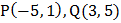# The sides of a triangle have the combined equation. The third side, which is variable always passes through the point. If the range of values of the slope of the third line is such that the origin is an interior point of the triangle isthen the value ofis

## Question ID - 153602 :- The sides of a triangle have the combined equation. The third side, which is variable always passes through the point. If the range of values of the slope of the third line is such that the origin is an interior point of the triangle isthen the value ofis

3537

(4)Nowlies onIn limiting case line passing throughcan be parallel toi.e.and maximum slope can occur if it passes through (0, 0)

i.e.andNext Question :
 The pointdivided the join ofin the ratio, then the integral value offor which the area ofwhereis (1, 5) andisis equal to 2 units in magnitude is